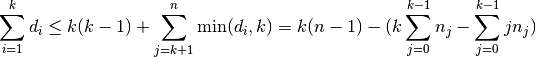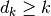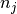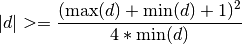Warning

This documents an unmaintained version of NetworkX. Please upgrade to a maintained version and see the current NetworkX documentation.

# is_valid_degree_sequence_erdos_gallai¶

is_valid_degree_sequence_erdos_gallai(deg_sequence)[source]

Returns True if deg_sequence can be realized by a simple graph.

The validation is done using the Erdős-Gallai theorem [EG1960].

Parameters: deg_sequence (list) – A list of integers valid – True if deg_sequence is graphical and False if not. bool

Notes

This implementation uses an equivalent form of the Erdős-Gallai criterion. Worst-case run time is: O(n) where n is the length of the sequence.

Specifically, a sequence d is graphical if and only if the sum of the sequence is even and for all strong indices k in the sequence,A strong index k is any index whereand the valueis the number of occurrences of j in d. The maximal strong index is called the Durfee index.

This particular rearrangement comes from the proof of Theorem 3 in .

The ZZ condition says that for the sequence d ifthen d is graphical. This was shown in Theorem 6 in .

References

  A. Tripathi and S. Vijay. “A note on a theorem of Erdős & Gallai”, Discrete Mathematics, 265, pp. 417-420 (2003).
  (1, 2) I.E. Zverovich and V.E. Zverovich. “Contributions to the theory of graphic sequences”, Discrete Mathematics, 105, pp. 292-303 (1992).